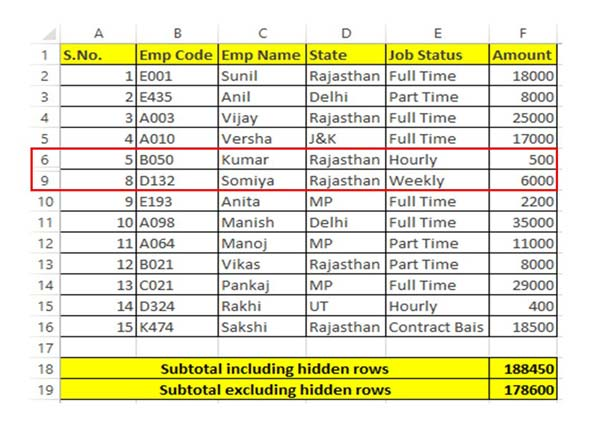# How to use Excel Subtotal Function

Excel Subtotal is an important function which is located in Math & Trig category which allows you to returns a subtotal in list or database.

Excel Subtotal function allows you to returns a subtotal in the list or database. Subtotal function in Excel return the Sum, Average, Count, Max, Min and many more. Excel Subtotal function is located in Math and Trig category. You can also use this function as a worksheet function in Excel.

There are two ways from which you can use Excel Subtotal function in active worksheet. You can either use Subtotal command from Data tab or you can use Subtotal function to get the result. Subtotal command from Data tab allows you to quickly calculate rows of related data by inserting subtotal and total in active worksheet.

### Syntax of Excel Subtotal Function

SUBTOTAL (function_num, ref1, [ref2], …)

function_num – It is required number between 1-11 or 101-111 that specifies which function to use in calculating subtotals within a list.

ref1 – This is required a named range or reference for which you want the subtotal.

ref2 – It is an optional argument. It is a named range or reference between 2-254 which you want the subtotal.

Subtotal is an important function which allows you to get a subtotal in a list or database. This function has ability to use different functions when subtotalling. You can use Average, Count, Max, Min, Sum and others in this function. You can also include or exclude the values in hidden rows.

When function_num is between 1 to 11, Subtotal function include all those cells which are hidden. When function_name is between 101 to 111, Subtotal function exclude values that are hidden.

## How to use Excel Subtotal Function

If you want to use Subtotal function in active worksheet then you have to take given few simple steps, have a look.Step 1: To understand how to use Subtotal function with or without hidden rows you have to create the given table structure . You can also open an existing table in which you want to use Subtotal function in Excel.

Step 2: After hide few rows from your database and want to include these hidden rows using Subtotal function, then you have to use given function. This function provide the sum of all rows which is given in the range including hidden rows.

=SUBTOTAL(9,F2:F16)

Step 3: If you want to hide few rows from your database and want to exclude these hidden rows using Subtotal function, then you have to use given function. This function provide the sum of all rows which is given in the range excluding hidden rows.

=SUBTOTAL(109,F2:F16)

Now, you can check both function result are different when you using Subtotal function in active worksheet.

I hope this guide helps you to use Subtotal function in active worksheet. If you have any query regarding this guide then please send us in the comment box. Thanks to all.